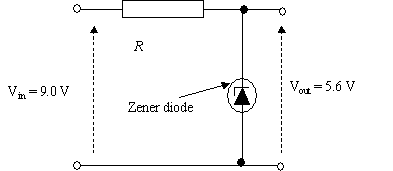# Topic 8 Operational AmplifiersThe operational amplifier was originally developed for analogue computing (our PCs are digital computers) and when introduced were complex and expensive components.  Now they are in integrated circuit form and are very cheap, about 50 p (0.7 Euros).  They are not very spectacular, but are extremely useful.

Operational amplifiers require a dual power supply, which means having a central 0 volts rail, and a + 15 V rail and a – 15 V rail.  The full circuit diagram is shown below, but generally we will ignore the power supply.

Notice that the op-amp has two inputs and one output.  It amplifies the difference between the inverting input and non-inverting input.  Be careful not to confuse the symbol with a non-inverting gate.

The ideal op-amp should have the following characteristics:

• Infinite open loop gain.
• Infinite input impedance so that no current is drawn.  (Impedance will covered in the next section)
• Zero output impedance so that maximum current can be transferred to the load.
• Very wide bandwidth.

Now try Question 1

In practice the maximum open loop gain is 200 000.  Beyond that limit the amplifier goes into saturation which means that the voltage cannot go any higher.  The voltage is limited, of course, by the supply voltages.  In practice the limits are rather lower than this, about 1.5 to 2 V below the value of the supply.

We can work out the input voltage that will give this swing.  Suppose the supply voltage was 15 V.  The maximum output voltage would be 15 – 1.5 = 13.5 V

So using a gain of 200 000:

Input voltage = ± 13.5 ÷ 200 000 = ± 67.5 mV

Therefore the input voltage can only swing through 135 mV in total to go from a negative saturation to a positive saturation.

The graph shows how the op-amp behaves:

Notice the following:

• The op-amp amplifies the difference between the two input voltages
• When the difference exceeds the limits X and Y, the output is saturated.
• In between the limits the graph is linear, so there is little distortion in these regions.

Now try Question 2

Using the Op-Amp as a Voltage Comparator

The op-amp being a differential amplifier is very useful as a voltage comparator.  It will give a high positive or negative voltage, depending on which voltage is higher:

• If the non-inverting input is higher, the output voltage will be positive
• If the inverting input is higher, the output voltage will be negative.
• The voltage difference must be greater than 135 mV if saturation is to occur.  This is not difficult to achieve.

The voltage comparator gives a digital output from an analogue input.

The diagram below shows the voltage comparator used as a light operated switch.

The diagram shows the voltage comparator used as a light operated switch.  This is how it works:

• The reference voltage is adjusted with the variable resistor so that it is equal to the voltage V2.
• When light falls on the LDR, V2 goes up because the resistance of the LDR goes down.
• V2 will be bigger than the reference voltage, so that the op-amp goes into saturation.
• The LED lights up, showing a high output.
• The reverse-biased diode protects the LED for when there is a large negative voltage.